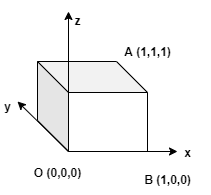# Problem: Find the angle between a diagonal of a cube and one of its edges.

###### FREE Expert Solution

We'll consider a cube of unit length:Vector OA = 1i + 1j + 1k

|OA| = (12 + 12 + 12)(1/2) = 3(1/2)

Vector OB = 1i + 0j + 0k

90% (434 ratings)###### Problem Details

Find the angle between a diagonal of a cube and one of its edges.